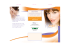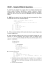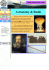# 15–122: Principles of Imperative Computation M E 1

## Transcription

15–122: Principles of Imperative Computation M E 1
```15–122: Principles of Imperative Computation
M IDTERM E XAM 1
S AMPLE S OLUTIONS
October 2, 2014
• This exam is closed book with one sheet of notes permitted.
• You have 80 minutes to complete the exam.
• There are 12 pages in this examination, comprising 4 questions worth a total of 125 points.
• Read each problem carefully before attempting to solve it.
• Do not spend too much time on any one problem.
• Consider if you might want to skip a problem on the first pass and return to it later.
Full Name:
Andrew ID:
Section Letter:
15–122 Midterm 1
1 of 12
October 9, 2014
Question:
Points:
True or False
Contracts
Pixels and Images
Spiral Sort
Total
31
37
27
30
125
Score:
15–122 Midterm 1
2 of 12
October 9, 2014
15–122 Midterm 1
3 of 12
October 9, 2014
Question 1 : True or False
(a) (21 points) For each of the following C0 statements, either a) write always true if the statement will always evaluate to true or b) give specific, concrete values of the variables in
either hex or in decimal such that the statement will either evaluate to false or raise an
arithmetic error.
x >> 2 == x / 4
x << 2 == x * 4
(~x) + 1 == -x
y <= 0 || (x / y) * y == x
x != -x
x != ~x
x <= x + 1
x any negative number not divisible
by 4
always true
always true
y positive and doesn’t evenly divide
x (example: x = 4, y = 3)
x is 0, 0x80000000, -231 , -2147483648
always true
x is 0x7FFFFFFF, 231 -1, 2147483647
(b) (10 points) Answer true or false (nothing more) to the following statements.
When creating an array in C0 with FALSE (the size can be 0)
alloc array, the size must be greater
than 0.
In the worst case, quicksort on an array of FALSE (average case
size n will run in O(n log n) time.
O(n log n), worst case
O(n2 ))
In the worst case, binary search in an array of TRUE
size n will run in O(log n) time.
3n + 4 ∈ O(n)
TRUE
n log n ∈ O(n)
FALSE
15–122 Midterm 1
4 of 12
October 9, 2014
Question 2 : Contracts
This function attempts to mimic the is_sorted(A, lower, upper) specification function.
It has all sorts of issues.
/* 1
/* 2
/* 3
/* 4
/* 5
/* 6
/* 7
/* 8
/* 9
/* 10
*/
*/
*/
*/
*/
*/
*/
*/
*/
*/
bool check_is_sorted(int[] A, int lower, int upper)
//@requires 0 <= lower && lower <= upper && upper <= \length(A);
{
for(int i = 0; i < upper; i++)
//@loop_invariant lower <= i && i <= upper;
{
if (A[i] > A[i+1]) return false;
}
return true;
}
If given the 5-element array containing the integers [1, 2, 3, 3, 2], as a first argument, give specific
values of lower and upper that meet the preconditions such that, when compiled and run with
contract checking on (-d). . .
(a) (6 points) . . . the function will return false without failing a contract or accessing an array out of bounds.
Answer: lower = 0, upper = 4
(b) (6 points) . . . the loop invariant will fail.
lower = 1, upper = 1,2,3,4,5
lower = 2, upper = 2,3,4,5
lower = 3, upper = 3,4,5
lower = 4, upper = 4,5
lower = 5, upper = 5
(c) (6 points) . . . an array will eventually be accessed out of bounds.
lower = 0, upper = 5 (the answer we’d originally intended)
“Something is wrong there’s no such counterexample” (actually correct answer)
(d) (6 points) Rewrite line 4 so that the loop invariant is valid, all array accesses are safe,
and the function correctly checks that the array is sorted from lower (inclusive) to upper
(exclusive).
for(int
for(int
for(int
for(int
15–122 Midterm 1
i
i
i
i
=
=
=
=
lower;
lower;
lower;
lower;
i < upper-1; i++)
i <= upper-2; i++)
i+1 < upper; i++) // Technically could overflow, w/e
i+2 <= upper; i++) // Technically could overflow, w/e
5 of 12
October 9, 2014
numbers. Do not list unnecessary line numbers.
/*
/*
/*
/*
/*
/*
/*
/*
/*
/*
/*
/*
/*
/*
1
2
3
4
5
6
7
8
9
10
11
12
13
14
*/
*/
*/
*/
*/
*/
*/
*/
*/
*/
*/
*/
*/
*/
int test(int[] A, int n)
//@requires 0 <= n;
//@requires n < \length(A);
{
int i = 0;
while (i < n)
//@loop_invariant 0 <= i;
//@loop_invariant i <= n;
{
A[i+1] = A[i+1] + 1;
i = i + 1;
}
return A[i] - 1;
}
(e) (3 points) Which line(s) would we need to reference to justify that the loop invariant
0 <= i on line 7 holds initially?
(f) (3 points) Which line(s) would we need to reference to justify that the loop invariant
i <= n on line 8 holds initially?
(g) (3 points) Which line(s) would we need to reference to justify the safety of the array accesses A[i+1] on line 10?
Answer: 7 (one point, lower bound) 6 and 3 (upper bound, two points)
(h) (4 points) Which line(s) would we need to reference to justify the safety of the array access A[i] on line 13?
Answer: Alternative 1: 2, 3, 6, and 8 (7 is unnecessary, we already know i == n)
Alternative 2: 3, 7, and 8
(7 tells us 0 <= i, and the other two tell use i <= n < \length(A), which suffices to
show safety).
Rubric:
3pts: 3, 6, 7, 8
3pts: 2, 3, 6, 7, 8
2pts: 3, 6, 8 or other “missing 1” answers
1pt: At least two of 2, 3, 6, 7, and 8
0pt: Use of lines 5, 10, or 11 at all
15–122 Midterm 1
6 of 12
October 9, 2014
Question 3 : Pixels and Images
Recall the interface to pixels, assuming a valid typedef for pixel:
pixel make_pixel(int a,
/*@requires 0 <= a && a
/*@requires 0 <= r && r
/*@requires 0 <= g && g
/*@requires 0 <= b && b
int
int
int
int
get_alpha(pixel P)
get_red(pixel P)
get_green(pixel P)
get_blue(pixel P)
int r,
< 256;
< 256;
< 256;
< 256;
int g, int b)
@*/
@*/
@*/
@*/ ;
/*@ensures
/*@ensures
/*@ensures
/*@ensures
0
0
0
0
<=
<=
<=
<=
\result
\result
\result
\result
&&
&&
&&
&&
\result
\result
\result
\result
<
<
<
<
256;
256;
256;
256;
@*/
@*/
@*/
@*/
;
;
;
;
(a) (3 points) The inversion transformation leaves alpha values of pixels untouched, but for
the red, green, and blue color intensities, it replaces an intensity of 255 with 0, an intensity
of 254 with 1, an intensity of 253 with 2... and so on to replacing an intensity of 0 with
255.
Implement the inversion transformation using only numeric constants written in hex and
the arithmetic operations from the set {+, -, * }. (You may not need to use all of them!)
pixel invert(pixel P) {
int a = get_alpha(P);
int r = get_red(P);
int g = get_green(P);
int b = get_blue(P);
return make_pixel(a, 0xFF-r, 0xFF-g, 0xFF-b);
}
(b) (3 points) Implement the inversion transformation using only numeric constants written
in hex and the bitwise operations from the set {~, ^, |, & }. (You may not need to use all
of them!)
pixel invert(pixel P) {
int a = get_alpha(P);
int r = get_red(P);
int g = get_green(P);
int b = get_blue(P);
return make_pixel(a, 0xFF^r, 0xFF^g, 0xFF^b);
}
15–122 Midterm 1
7 of 12
October 9, 2014
(c) (11 points) Given the following struct declaration and typedefs, fill in a data structure
invariant is_pixel and implement make_pixel and get_red. All functions should be
safe, correct, and should provably satisfy their contracts.
int alpha; // Stores the alpha value
int red;
// Stores the red value
int green; // Stores the green value
int blue; // Stores the blue value
};
bool is_pixel(____pixel or struct pixel_header*____ P) {
if (P == NULL) return false;
if (!(0 <= P->alpha && P->alpha < 256)) return false;
if (!(0 <= P->red && P->red < 256)) return false;
if (!(0 <= P->green && P->green < 256)) return false;
if (!(0 <= P->blue && P->blue < 256)) return false;
return true;
}
pixel make_pixel(int a, int r, int g, int b)
//@requires 0 <= a && a < 256 && 0 <= r && r < 256;
//@requires 0 <= g && g < 256 && 0 <= b && b < 256;
//@ensures is_pixel(\result);
{
P->alpha = a;
P->red = r;
P->green = g;
P->blue = b;
return P;
}
int get_red(pixel P)
//@requires is_pixel(P);
//@ensures 0 <= \result && \result < 256;
{
return P->red;
}
15–122 Midterm 1
8 of 12
October 9, 2014
(d) (4 points) This alternate implementation of pixels ADT is safe, and it provably satisfies
its contracts, but it’s not correct.
bool is_pixel(/* SAME TYPE AS BEFORE (NOT SHOWN) */ P) {
return true;
}
pixel make_pixel(int a, int r, int g, int b)
//@requires 0 <= a && a < 256 && 0 <= r && r < 256;
//@requires 0 <= g && g < 256 && 0 <= b && b < 256;
//@ensures is_pixel(\result);
{
return NULL;
}
int get_red(pixel P)
//@requires is_pixel(P);
//@ensures 0 <= \result && \result < 256;
{
return 200;
}
... other functions not shown
Write a simple unit test given the code above that respects the pixel interface and detects the
bug in this implementation by failing an assertion.
int main() {
pixel P = make_pixel(0, 0, 0, 0);
assert(get_red(P) == 0);
return 0;
}
15–122 Midterm 1
9 of 12
October 9, 2014
(e) (6 points) Consider two versions of the same image, which has width of w and height of
h. The first is an arbitrary image, and the second is a version of the original image where
each row has been sorted by average pixel intensity. That is, the average of the red, green
and blue values is computed for each pixel, and the row of pixels is sorted based on these
average values. Here’s one example of such a pair of images:
Using selection sort, the time it would take to produce the image on the right from the
image on the left would be in O(hw2 ), where h is the height and w is the width. If this
process took exactly 1 second on an image with height 500 and width 500, approximately
how long would we expect this sorting process to take if the height was 1500 and the
width was 2000?
Using the sorted image as described above and any algorithms discussed in class, how
long would it take to efficiently determine whether a pixel with an average intensity i
exists anywhere in the image? Give your answer using big O notation in terms of w and
h, in its tightest, simplest form.
15–122 Midterm 1
10 of 12
October 9, 2014
Question 4 : Spiral Sort
In this problem, we discuss spiral sort. Its chief (and perhaps its only) virtue is that its code is
exceedingly short.
/*
/*
/*
/*
/*
/*
/*
/*
/*
/*
/*
/*
/*
/*
/*
1
2
3
4
5
6
7
8
9
10
11
12
13
14
15
*/
*/
*/
*/
*/
*/
*/
*/
*/
*/
*/
*/
*/
*/
*/
void spiralsort(int[] A, int n)
//@requires 0 <= n && n <= \length(A);
//@ensures is_sorted(A, 0, n);
{
for (int i = 0; i < n; i++)
//@loop_invariant 0 <= i && i <= n;
//@loop_invariant is_sorted(A, 0, i);
for (int k = 0; k < i; k++)
//@loop_invariant 0 <= k && k <= i;
//@loop_invariant is_sorted(A, 0, i);
// Another loop invariant will be needed...
if (A[i] < A[k])
swap(A, i, k);
return;
}
The loop invariants given above will never fail during the actual evaluation of spiralsort,
but the loop invariants on the inner loop are not strong enough to prove the correctness of the
(a) (4 points) What is the worst-case running time of spiral sort on an array of length n, using
big-O notation? (When compiled without -d, of course.)
(b) (5 points) Show that we can’t reason about the inner loop invariant being preserved: assuming i = 3, give a value for k and contents of an array A such that the loop invariants
on lines 9 and 10 hold, the loop guard on line 8 evaluates to true, but the loop invariant
on line 10 will not hold the next time it is checked.
i = 3
k = either 1 or 2
A = [1,2,3,0,whatever] – A must be less than BOTH A[k] and A[k-1]
15–122 Midterm 1
11 of 12
October 9, 2014
(c) (5 points) The loop invariant A[k-1] <= A[i] is almost right. What would be wrong with
adding this as a loop invariant on line 11?
Answer: If k == 0 this will cause an array-out-of-bounds access.
(d) (6 points) Give a better additional loop invariant for the inner loop (which would belong
on line 11) that allows us to show that all loop invariants are preserved. You can use
functions from arrayutil.c0 as discussed in class, but this is not necessary.
k == 0 || A[k-1] <= A[i]
or ge_seg(A[i], A, 0, k)
(e) (10 points) Taking for granted that the inner loop invariants are true initially and preserved by every iteration of the loop, show concisely why the outer loop invariants are
preserved by every iteration of the outer loop. Specify line numbers to make your reasoning easier to follow. You’ll need to use your answer in part (d) at some point.
Preservation of loop invariant 0 <= i && i <= n
Need to show: 0 <= i’ && i’ <= n
By line 5, i’ is i+1, so we need to show 0 <= i+1 and i+1 <= n.
We know 0 <= i+1 because 0 <= i (line 6).∗
We know i+1 <= n because the loop guard (line 5) tells us i < n.
(Worth 4 points.)
∗ To complete this argument, we really ought to add that because i < n (loop guard
on line 5) we know i+1 doesn’t overflow, so i < i+1.
Preservation of loop invariant is sorted(A, 0, i)
Need to show: is sorted(A, 0, i’)
By line 5 i’ is i+1, so we need to show is_sorted(A, 0, i+1).
We know k == i by the negation of the loop guard and loop invariant (lines 8&9).
We therefore know, by substitution and line 6, that A[i] >= A[0,i).
Combined with is_sorted(A, 0, i) (line 10), this means is_sorted(A, 0, i+1).
(Worth 6 points)
15–122 Midterm 1
12 of 12
October 9, 2014
```

### 15-122 : Principles of Imperative Computation, Spring 2013 Homework 2 Theory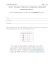### How to install RCS/RK TAGHeuer By Chronelec active loop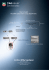### NIS-Elements: How to use Pixel Classifier to produce an Area Fraction... Pixel Classifier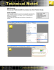### TEMPLATE HOW-TO MAKE A GUM PASTE BOW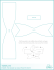### XMPlayer Express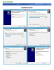### ICSE Computer Application Sample Papers SECTION A (40 Marks)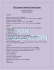### Sample Midterm CSE 680: Introduction to Algorithms and Data Structures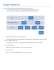### Pixel Perfect